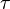Hello,

I am trying to analyze a bolted connection. I don't remember anything about bolt connections, so I am studying at the same time ( the purpose is to actually study before actually designing a bolted connection). I designed the system itself and found the stresses on the connection and trying to understand whether the current bolt system is enough to support it.

Here is the problem: The bolted connection has 8 M20 bolts, 4 at the left hand side and 4 at the right hand side, so not symmetrical. The connection is under all kinds of forces and bending moments, so Fx, Fy, Fz and Mx, My, and Mz are all present (x being the direction perpendicular to the cross section). The stresses due to bending moments are where I am stuck.

Here is what I did so far:

I assumed only the four bolts at the corners are carrying the loads so that the arrangement becomes symmetrical for simplicity. For example, the bending moment in the y direction is 7500000 Nmm. I carried this bending moment to the centroid. The distance between a bolt and the centroid is about 150 mm. So the summation of moments on each bolt should be equal to the actual bending moment. Is that a correct procedure (this is what I get from the textbook)?

7500000/4=1875000 Nmm (4 bolts assumed)
1875000/150=12500 N (Force causing the bending moment)
12500N/245=51 MPa tensile stress (245 mm being the tensile stress area of a M20 bolt).

Similarly in z direction (11200000 Nmm),

112000000/4=2800000 Nmm
2800000/150=18667 N
18667/245=76 MPa tensile stress

And how should I proceed in the x direction? This method wouldn't work and I haven't come up with something yet.

Thanks for any help.

Last edited:

Hello,

I am trying to analyze a bolted connection. I don't remember anything about bolt connections, so I am studying at the same time ( the purpose is to actually study before actually designing a bolted connection). I designed the system itself and found the stresses on the connection and trying to understand whether the current bolt system is enough to support it.

Here is the problem: The bolted connection has 8 M20 bolts, 4 at the left hand side and 4 at the right hand side, so not symmetrical. The connection is under all kinds of forces and bending moments, so Fx, Fy, Fz and Mx, My, and Mz are all present (x being the direction perpendicular to the cross section). The stresses due to bending moments are where I am stuck.

Here is what I did so far:

I assumed only the four bolts at the corners are carrying the loads so that the arrangement becomes symmetrical for simplicity. For example, the bending moment in the y direction is 7500000 Nmm. I carried this bending moment to the centroid. The distance between a bolt and the centroid is about 150 mm. So the summation of moments on each bolt should be equal to the actual bending moment. Is that a correct procedure (this is what I get from the textbook)?

7500000/4=1875000 Nmm (4 bolts assumed)
1875000/150=12500 N (Force causing the bending moment)
12500N/245=51 MPa tensile stress (245 mm being the tensile stress area of a M20 bolt).

Similarly in z direction (11200000 Nmm),

112000000/4=2800000 Nmm
2800000/150=18667 N
18667/245=76 MPa tensile stress

And how should I proceed in the x direction? This method wouldn't work and I haven't come up with something yet.

Thanks for any help.
Can you please provide figure for the same. Its difficult to understand this way.

Even hand drawn photo will be much better..

I attached the picture, this is the bolt arrangement. Sorry I am in a hurry and just inserted the axes in paint haha. I made up the dimensions in the first post but this is the actual system. I performed an analysis and found the support reactions in the system, Fx, Fy, Fz, Mx, My, Mz. Trying to find out if the bolt arrangement is enough to support these support reactions.There is also another attached 3d pic of the actual system.

#### Attachments

•Bolts.PNG
74.9 KB · Views: 1,977
PhanthomJay
Homework Helper
Gold Member

•George Zucas
Thanks a lot PhantomJay. I made up the numbers in the original post but I added the actual results from the analysis now (Theser are the support reaction forces and moments I get). Anyway, the problem is much clearer to me now. I'll try to solve every kind of stress tomorrow and post it if it is correct (well I am almost asleep:) ).

#### Attachments

This is my solution so far:

Mz=12631221 Nmm
sigma=((12631221*100)/(80000*245))=64.45 MPa on each bolt.

----

My=6502447 Nmm
Coner bolts to y: 90 mm
Inbetween bolts to y axis: 30 mm
For the center bolts: sigma=(6502447*90)/(36000*245)=66.35 MPa on each bolt
For the mid bolts: sigma= (6502447*30)/(36000*245)=22.12 MPa on each bolt

----

Mx=5265769 Nmm
Total J=4*(sqrt(90^2+100^2))^2+4*(sqrt(30^2+100^2))^2=116000 mm^2
Corner bolts to centroid: 134.5 mm
Mid bolts to centroid: 104.4 mm

Corner bolts:= (5265769*134.5)/(116000*225)=27.14 MPa on each bolt (225mm2 shear stress area of the bolt)
Mid bolts:=(5265769*104.5)/(116000*225)=21.08 MPa

So for the critical corner bolts
Total Tensile Stress= 130.8 MPa
Total Shear Stress=27.14 MPa

Does this look correct (fingers crossed)?

PhanthomJay
Homework Helper
Gold Member
yes looks very good. Now you have to add in the contributions from the Fx , Fy , and Fz loads.

Last edited by a moderator:
•George Zucas
yes looks very good. Now you have to add in the contributions from the Fx , Fy , and Fz loads.

Great. I think I got the forces down, just divide the forces by the total bolt area, which is total tensile stress area for the tensile x load and shear stress area for the shear y and z loads.

Thanks a lot, can't express how much I appreciated your help.

PhanthomJay
•